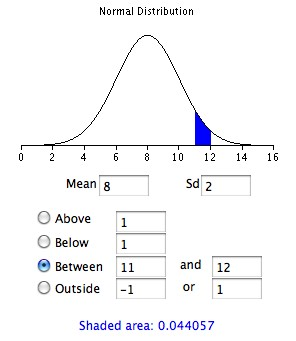# The Weight Of Items Produced By A Machine Is Normally Distributed With A Mean Of 8 Ounces And A Standard Deviation Of 2 Ounces. Refer To Exhibit 6-5. What Is The Probability That A Randomly Selected Item Will Weigh Between 11 And 12 Ounces?

Unfortunately, without the exhibit 6-5 obtaining an answer is difficult.

To answer this question more information would be needed. Obviously it has been stated that an average piece of 8 ounces is generally produced on a machine. We also know the standard deviation is 2 ounces; however, the exhibit 6-5 indicates there is more information that would help in deriving the probability of getting a weight between 11 and 12 ounces.

• How to find the answer

You may also need to study a statistics textbook in how to derive probability. According to this site www.statisticshowto.com/articles/finding-the-probability-of- you need to have the percentage of certain numbers. You will then turn the percentage into a decimal.

In the next step you take the decimal and multiply it by the number of times you have that percentage appear. If you do not have a percentage you will first have to find out what it might be and then add the probabilities you find together.

For example if the table tells you that to obtain 2 ounces there is a .25 chance of that happening and you have a .50 chance of obtaining 8 ounces then you could infer that the probability of 11 to 12 ounces would be .25 or 25 percent probable. It would just depend on how many other probabilities you have to figure into the issue.

This is why the table or exhibit is needed to determine your answer, as it should have the information needed to do the appropriate calculation. Keep in mind that all probabilities total to 100 percent.
thanked the writer.